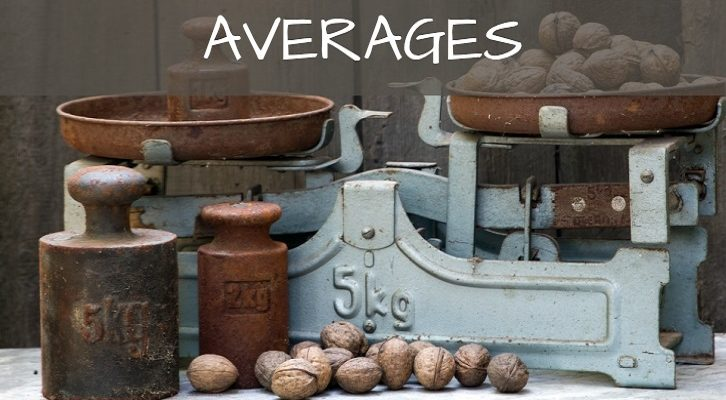# Mean Median (Averages) – Solutions

Have given below the solutions to the questions on mean and median .

1. Consider 4 numbers a, b, c and d. Ram figures that the smallest average of some three of these four numbers is 30 and the largest average of some three of these 4 is 40. What is the range of values the average of all 4 numbers can take?

We can assume a, b,c d are in ascending order (with the caveat that numbers can be equal to each other)

a + b + c = 90

b + c + d = 120

We need to find the maximum and minimum value of a + b + c + d.

a + b + c + d = 120 + a. So, this will be minimum when a is minimum. Given a + b + c = 90. a is minimum when b + c is maximum. If b + c is maximum, d should be minimum. Given that b + c + d = 120, the minimum value d can take is 40 as d cannot be less than b or c. The highest value b +c can take is 80, when b = c= d = 40. When b = c = d = 40, a = 10. a + b + c + d = 130. Average = 32.5

Similarly, a + b + c + d = 90 + d. So, this will be maximum when d is maximum. Given b + c + d = 120. d is maximum when b + c is minimum. If b + c is minimum, a should be maximum. Given that a + b + c = 90, the maximum value a can take is 30 as a cannot be greater than b or c. The lowest value b +c can take is 60, when a = b= c = 30. When a = b = c = 30, d = 60. a + b + c + d = 150. Average = 37.5

So, the average has to range from 32.5 to 37.5

2. The average of 5 distinct positive integers if 33. What are the maximum and minimum possible values of the median of the 5 numbers if the average of the three largest numbers within this set is 39?

Let the numbers be a, b, c, d, e in ascending order. a + b + c + d + e = 165. Average of the three largest numbers is 39, so c + d + e = 117, or a + b = 48.

We need to find the maximum and minimum possible values of c.

For minimum value, a and b have to be minimum. a +b = 48. Let us assume a =23, b =25, ca can be as low as 26.

23, 25, 26, 45, 46 is a possible sequence that satisfies the conditions.

For maximum value, we need d + e to be minimum as c + d + e = 117. we can have c = 38, d =39 and e =40. Or, the maximum value c can take = 38

23, 25, 38, 39, 40 is a possible sequence that satisfies the conditions specified

3. Consider 5 distinct positive numbers a, b, c, d, and e. The average of these numbers is k. If we remove b from this set, the average drops to m (m is less than k). Average of c, b, d and e is K. We also know that c is less than d and e is less than k. The difference between c and b is equal to the difference between e and d. Average of a, b, c and e is greater than m. Write down a, d, c, d and e in ascending order.

Average of a, b, c, d and e is k, Average of b, c, d and e is also k, this implies that a = k

If we remove b, the average drops, this implies that b is higher than the average

e is less than k, or e is less than a. c is less than d.

e < a < b, c < d

Average of a, b, c and e is greater than average a, c, d and e. This tells us that d < b

From this, we get that b is the largest number

b – c = d – e

b + e = c + d

a + b + c +d + e = 5a

Or, b + c + d + e = 4a

b + e = 2a, c + d = 2a. Or, e, a, b is an AP, c, a, d is an AP.

e is the smallest number (as b is the largest number)

Or, the ascending order should be e c a d b